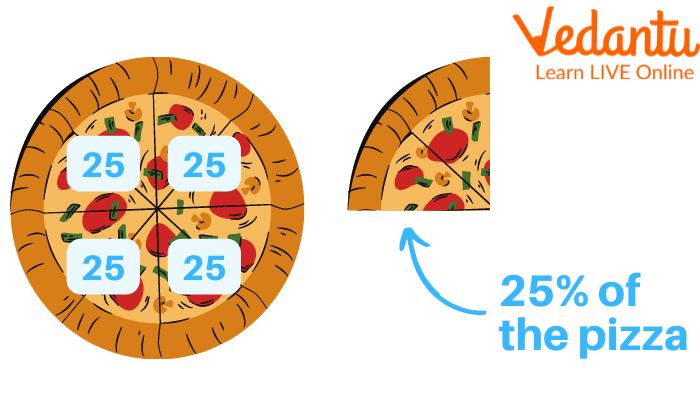Courses
Courses for Kids
Free study material
Free LIVE classes
More

# How to Convert Ratio into Percentage?## Introduction to Ratio

Last updated date: 18th Mar 2023
Total views: 58.2k
Views today: 0.37k

In maths, a ratio shows how many times one number contains another number, or can say that ratio is the comparison of numbers? Suppose there are two numbers a and b. In ratios, the ratio of number a to number b is often written as a:b ‘:' is the sign of ratios.

For example- Neha's weight is 40 kg and her mother's weight is 60 kg. So the ratio of Neha's mother's weight To Neha's weight will be $\dfrac{60}{40} = \dfrac{3}{2}$ = 3:2. This Ratio can be converted into percentages just by using a simple formula. Ratios are converted into percentages to make us easy to understand.Image Showing Ratio of Boys to Girls.

## What is the Percentage?

In maths, we can say that a percentage is a number expressed as a fraction of a hundred. It is often denoted by the '%' sign.

## How to Convert Ratio into Percentage?

Ratios can be converted into percentages in different ways. These ratios can be converted into normal percentages, fraction percentages, and decimal percentages. Let us understand how to convert each part of the ratio into a percentage.Image Showing Part of Pizza as a Percentage.

There is a basic formula for converting ratios into percentages. This formula can be used with any value Percentage Formula = ( Ratio × 100 ) %

Now let's understand the method of converting each part of the ratios into percentages in detail.

To convert the ratio into a percentage.

• Step 1. Obtain the given ratio in the form of x:y

• Step 2. Convert the above ratio into a fraction such as $\dfrac{x}{y}$

• Step 3. See if you can further simplify the given fraction.

• Step 4. Multiply the given fraction by 100 and put the percentage sign (%)

For example- Let the given ratio be 3:4

Ans: Let us understand the percentage of 3:4. On converting the given ratio into a fraction 3:4 = $\dfrac{3}{4}$

On multiplying the obtained fraction by 100.

$\dfrac{3}{4} \times 100$ % = 75 %

Therefore, the percentage of 3:4 is 75%

Example: Convert $\dfrac{21}{15}$ into a percentage

Ans. On converting into fraction 21:15 = $\dfrac{21}{15}$

On simplify

$\dfrac{21}{15}$ = $\dfrac{7}{5}$

On multiplying by 100.

$\dfrac{7}{5} \times 100$ %= 140%

## To Convert the Given Ratio into a Fraction Percentage

Sometimes the given ratio cannot be fully converted into a percentage so convert it into a fraction percentage.

Example 1: Convert 3:14 into a percentage.

Ans. Let us understand how to convert 3:14 by using a ratio to percentage formula. On converting the ratio into fraction 3:14 = $\dfrac{3}{14}$

Now on multiplying the given fraction by 100.

$\dfrac{3}{14} \times 100$ % = $\dfrac{150}{7}\%$

Therefore, 3:4 into fraction percentage is $\dfrac{150}{7}\%$

Example 2: Convert 6:36 into a percentage.

Ans. On converting it into fraction 6:36 = $\dfrac{6}{36}$

If the given numerator and denominator have the same factors, they can be further simplified by curing.

On simplification

$\dfrac{6}{36}$ = $\dfrac{1}{6}$

On multiplication by 100

$\dfrac{1}{6} \times 100$ % =$\dfrac{50}{3}\%$

Therefore 6:36 into percentage is $\dfrac{50}{3}\%$.

## How To Convert a Given Ratio into a Decimal Percentage

Let us convert 14:16 into a decimal percentage

On converting into a fraction

14:16 = $\dfrac{14}{16}$

On simplification

$\dfrac{14}{16}$ = $\dfrac{7}{8}$

On multiplication by 100

$\dfrac{7}{8} \times 100$ % =$\dfrac{175}{2}\%$

And on further dividing

$\dfrac{175}{2}$ = 87.5 %

Example: Convert 7:6 into a decimal percentage.

Ans. Converting the given ratio into fraction 7:6= $\dfrac{7}{6}$

On multiplying the given number by 100

( $\dfrac{7}{6}$ × 100 ) % = $\dfrac{350}{3}\%$

On further dividing $\dfrac{350}{3}$ = 116.67 %

## Practice Questions

Q1. Convert the following ratios into percentages.

1.16:25

Ans: 64%

2. 10:25

Ans: 40%

Q2. Convert the following ratios into a fraction and decimal percentage

1. 8:15

Ans: $\dfrac{160}{3}\%$ or 53.34%

2. 4:75

Ans: $\dfrac{16}{3}\%$ or 5.34%

## Summary

The ratio shows how many times one number contains another number. It is denoted by the ': ' sign. The ratio of two numbers a and b is a:b. A percentage is a number expressed as a fraction of a hundred. It is often denoted by the '%' sign. Ratio and percentage help us to understand numbers better. These ratios can be converted into a normal percentage, fraction percentage, and decimal percentage. The formula to convert the given ratio into a percentage is ( Ratio × 100 ) %.

## FAQs on How to Convert Ratio into Percentage?

1. How are percentages used in everyday life?

Percentages are simplified ways of expressing size, scale, or value. They are used in many different areas of everyday life. For example- when you visit a mall you can see discounts on various clothes, accessories, electronic gadgets, coupons, etc. Bank interest rates, and inflation rates are also in percentage.

2. How are ratios used in everyday life?

Ratios allow us to measure quantities, they also help us to understand the quantities easily. There are many real-life uses of ratios. Some of them are as follows-

Ratios allow cooks to use the measurements of ingredients correctly. Many recipes need ingredients to be used in proportion. The ratio also helps people in the business world.

3. How are percentages and ratios different?

The ratio is converted to percentages to make us understand maths in a better way. The ratio is used to compare two numbers in the same unit. At the same time, percentages are specific types of ratios in which the value of the whole is always equal to 100. In ratios instead of comparing two parts of the whole against each other, percentages compare any one part against the whole. Percentages are better for comparisons of data sets of different sizes.